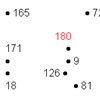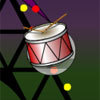# Search by Topic

#### Resources tagged with Factors and multiples similar to Room Doubling:

Filter by: Content type:
Age range:
Challenge level:

### There are 142 results

Broad Topics > Numbers and the Number System > Factors and multiples### It Figures

##### Age 7 to 11 Challenge Level:

Suppose we allow ourselves to use three numbers less than 10 and multiply them together. How many different products can you find? How do you know you've got them all?### The Moons of Vuvv

##### Age 7 to 11 Challenge Level:

The planet of Vuvv has seven moons. Can you work out how long it is between each super-eclipse?### A Mixed-up Clock

##### Age 7 to 11 Challenge Level:

There is a clock-face where the numbers have become all mixed up. Can you find out where all the numbers have got to from these ten statements?### Mystery Matrix

##### Age 7 to 11 Challenge Level:

Can you fill in this table square? The numbers 2 -12 were used to generate it with just one number used twice.### Neighbours

##### Age 7 to 11 Challenge Level:

In a square in which the houses are evenly spaced, numbers 3 and 10 are opposite each other. What is the smallest and what is the largest possible number of houses in the square?### Multiplication Squares

##### Age 7 to 11 Challenge Level:

Can you work out the arrangement of the digits in the square so that the given products are correct? The numbers 1 - 9 may be used once and once only.### Multiples Grid

##### Age 7 to 11 Challenge Level:

What do the numbers shaded in blue on this hundred square have in common? What do you notice about the pink numbers? How about the shaded numbers in the other squares?### Seven Flipped

##### Age 7 to 11 Challenge Level:

Investigate the smallest number of moves it takes to turn these mats upside-down if you can only turn exactly three at a time.### Sweets in a Box

##### Age 7 to 11 Challenge Level:

How many different shaped boxes can you design for 36 sweets in one layer? Can you arrange the sweets so that no sweets of the same colour are next to each other in any direction?### Two Primes Make One Square

##### Age 7 to 11 Challenge Level:

Can you make square numbers by adding two prime numbers together?### Sets of Numbers

##### Age 7 to 11 Challenge Level:

How many different sets of numbers with at least four members can you find in the numbers in this box?### Abundant Numbers

##### Age 7 to 11 Challenge Level:

48 is called an abundant number because it is less than the sum of its factors (without itself). Can you find some more abundant numbers?### A Dotty Problem

##### Age 7 to 11 Challenge Level:

Starting with the number 180, take away 9 again and again, joining up the dots as you go. Watch out - don't join all the dots!### Add the Weights

##### Age 7 to 11 Challenge Level:

If you have only four weights, where could you place them in order to balance this equaliser?### Pebbles

##### Age 7 to 11 Challenge Level:

Place four pebbles on the sand in the form of a square. Keep adding as few pebbles as necessary to double the area. How many extra pebbles are added each time?### Tiling

##### Age 7 to 11 Challenge Level:

An investigation that gives you the opportunity to make and justify predictions.### Multiply Multiples 2

##### Age 7 to 11 Challenge Level:

Can you work out some different ways to balance this equation?### Fractions in a Box

##### Age 7 to 11 Challenge Level:

The discs for this game are kept in a flat square box with a square hole for each. Use the information to find out how many discs of each colour there are in the box.### Curious Number

##### Age 7 to 11 Challenge Level:

Can you order the digits from 1-3 to make a number which is divisible by 3 so when the last digit is removed it becomes a 2-figure number divisible by 2, and so on?### Multiply Multiples 1

##### Age 7 to 11 Challenge Level:

Can you complete this calculation by filling in the missing numbers? In how many different ways can you do it?### Ip Dip

##### Age 5 to 11 Challenge Level:

"Ip dip sky blue! Who's 'it'? It's you!" Where would you position yourself so that you are 'it' if there are two players? Three players ...?### Multiply Multiples 3

##### Age 7 to 11 Challenge Level:

Have a go at balancing this equation. Can you find different ways of doing it?### A Square Deal

##### Age 7 to 11 Challenge Level:

Complete the magic square using the numbers 1 to 25 once each. Each row, column and diagonal adds up to 65.### Gran, How Old Are You?

##### Age 7 to 11 Challenge Level:

When Charlie asked his grandmother how old she is, he didn't get a straightforward reply! Can you work out how old she is?### Zios and Zepts

##### Age 7 to 11 Challenge Level:

On the planet Vuv there are two sorts of creatures. The Zios have 3 legs and the Zepts have 7 legs. The great planetary explorer Nico counted 52 legs. How many Zios and how many Zepts were there?### Ben's Game

##### Age 11 to 14 Challenge Level:

Ben passed a third of his counters to Jack, Jack passed a quarter of his counters to Emma and Emma passed a fifth of her counters to Ben. After this they all had the same number of counters.### Crossings

##### Age 7 to 11 Challenge Level:

In this problem we are looking at sets of parallel sticks that cross each other. What is the least number of crossings you can make? And the greatest?### Fitted

##### Age 7 to 11 Challenge Level:

Nine squares with side lengths 1, 4, 7, 8, 9, 10, 14, 15, and 18 cm can be fitted together to form a rectangle. What are the dimensions of the rectangle?### Sets of Four Numbers

##### Age 7 to 11 Challenge Level:

There are ten children in Becky's group. Can you find a set of numbers for each of them? Are there any other sets?### A First Product Sudoku

##### Age 11 to 14 Challenge Level:

Given the products of adjacent cells, can you complete this Sudoku?### What Do You Need?

##### Age 7 to 11 Challenge Level:

Four of these clues are needed to find the chosen number on this grid and four are true but do nothing to help in finding the number. Can you sort out the clues and find the number?### Making Pathways

##### Age 7 to 11 Challenge Level:

Can you find different ways of creating paths using these paving slabs?### Scoring with Dice

##### Age 7 to 11 Challenge Level:

I throw three dice and get 5, 3 and 2. Add the scores on the three dice. What do you get? Now multiply the scores. What do you notice?### How Old Are the Children?

##### Age 11 to 14 Challenge Level:

A student in a maths class was trying to get some information from her teacher. She was given some clues and then the teacher ended by saying, "Well, how old are they?"### Number Detective

##### Age 5 to 11 Challenge Level:

Follow the clues to find the mystery number.### Cuisenaire Environment

##### Age 5 to 11 Challenge Level:

An environment which simulates working with Cuisenaire rods.### Being Resilient - Primary Number

##### Age 5 to 11 Challenge Level:

Number problems at primary level that may require resilience.### Being Collaborative - Primary Number

##### Age 5 to 11 Challenge Level:

Number problems at primary level to work on with others.### Factors and Multiples Game for Two

##### Age 7 to 14 Challenge Level:

Factors and Multiples game for an adult and child. How can you make sure you win this game?### American Billions

##### Age 11 to 14 Challenge Level:

Play the divisibility game to create numbers in which the first two digits make a number divisible by 2, the first three digits make a number divisible by 3...### Which Is Quicker?

##### Age 7 to 11 Challenge Level:

Which is quicker, counting up to 30 in ones or counting up to 300 in tens? Why?### Factor-multiple Chains

##### Age 7 to 11 Challenge Level:

Can you see how these factor-multiple chains work? Find the chain which contains the smallest possible numbers. How about the largest possible numbers?### Divide it Out

##### Age 7 to 11 Challenge Level:

What is the lowest number which always leaves a remainder of 1 when divided by each of the numbers from 2 to 10?### Multiplication Square Jigsaw

##### Age 7 to 11 Challenge Level:

Can you complete this jigsaw of the multiplication square?### Beat the Drum Beat!

##### Age 7 to 11 Challenge Level:

Use the interactivity to create some steady rhythms. How could you create a rhythm which sounds the same forwards as it does backwards?### Which Numbers? (2)

##### Age 7 to 11 Challenge Level:

I am thinking of three sets of numbers less than 101. Can you find all the numbers in each set from these clues?### Which Numbers? (1)

##### Age 7 to 11 Challenge Level:

I am thinking of three sets of numbers less than 101. They are the red set, the green set and the blue set. Can you find all the numbers in the sets from these clues?### Becky's Number Plumber

##### Age 7 to 11 Challenge Level:

Becky created a number plumber which multiplies by 5 and subtracts 4. What do you notice about the numbers that it produces? Can you explain your findings?### Three Dice

##### Age 7 to 11 Challenge Level:

Investigate the sum of the numbers on the top and bottom faces of a line of three dice. What do you notice?### Give Me Four Clues

##### Age 7 to 11 Challenge Level:

Four of these clues are needed to find the chosen number on this grid and four are true but do nothing to help in finding the number. Can you sort out the clues and find the number?